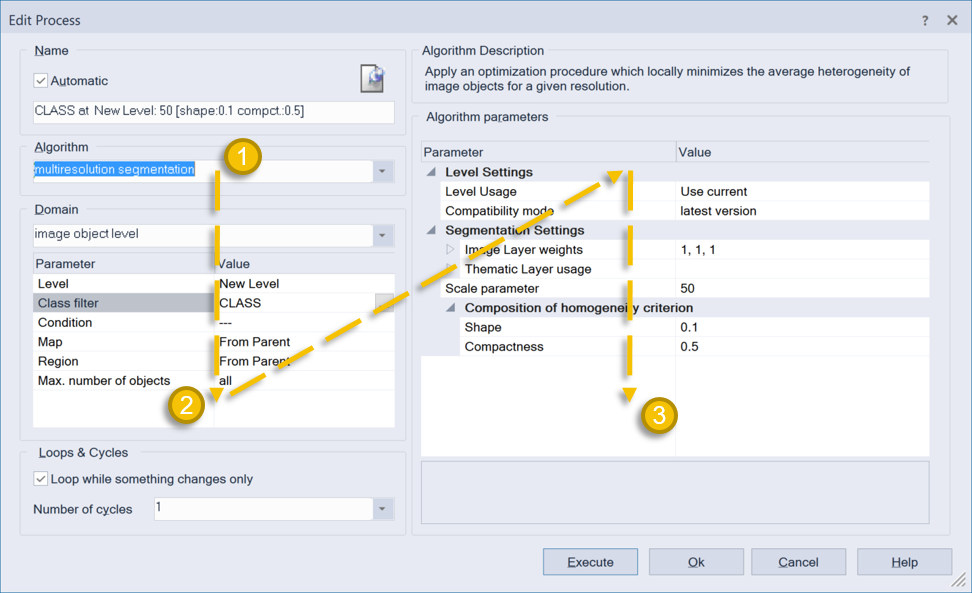The Edit Process window is an elementary part of rule set development in eCognition. Therefore, it is important to understand how this window functions for efficient rule set creation and algorithm configuration.

If you open the Edit Process window from the Process Tree, you will notice that it is split into 3 general sections. We like to think of these sections as describing the “what”, “where” and “how” of CNL. The best way to navigate through the window is in a reverse N-like motion: ↓↗↓ (see graphic below).

1. The Algorithm section is the “what” – as in what algorithm will be applied? Here the user can use the drop-down menu to select an algorithm. Alternatively, you can begin typing the name of the algorithm and the search results will be narrowed down. In this example, the Multiresolution Segmentation algorithm is selected.
2. The Domain section is the “where” – as in where will this algorithm be applied (i.e. on the pixel level or image object level)? Here the user can define if the algorithm should be restricted to consider a specific map or image objects based on a class filter and/or feature criteria. In this example, the segmentation will only be applied to image objects with the class name “CLASS”.
3. The Algorithm parameters section is the “how” - as how should the algorithm be applied? Here the user can adjust various algorithm parameters to focus output on a specific use case. In this example, the user has options for adjusting the segmentation to account for specific input layers as well as parameters that impact the size and shape of the objects that will be created (i.e. scale parameter, shape & compactness).